SQL Having Clause Example | SQL Having Tutorial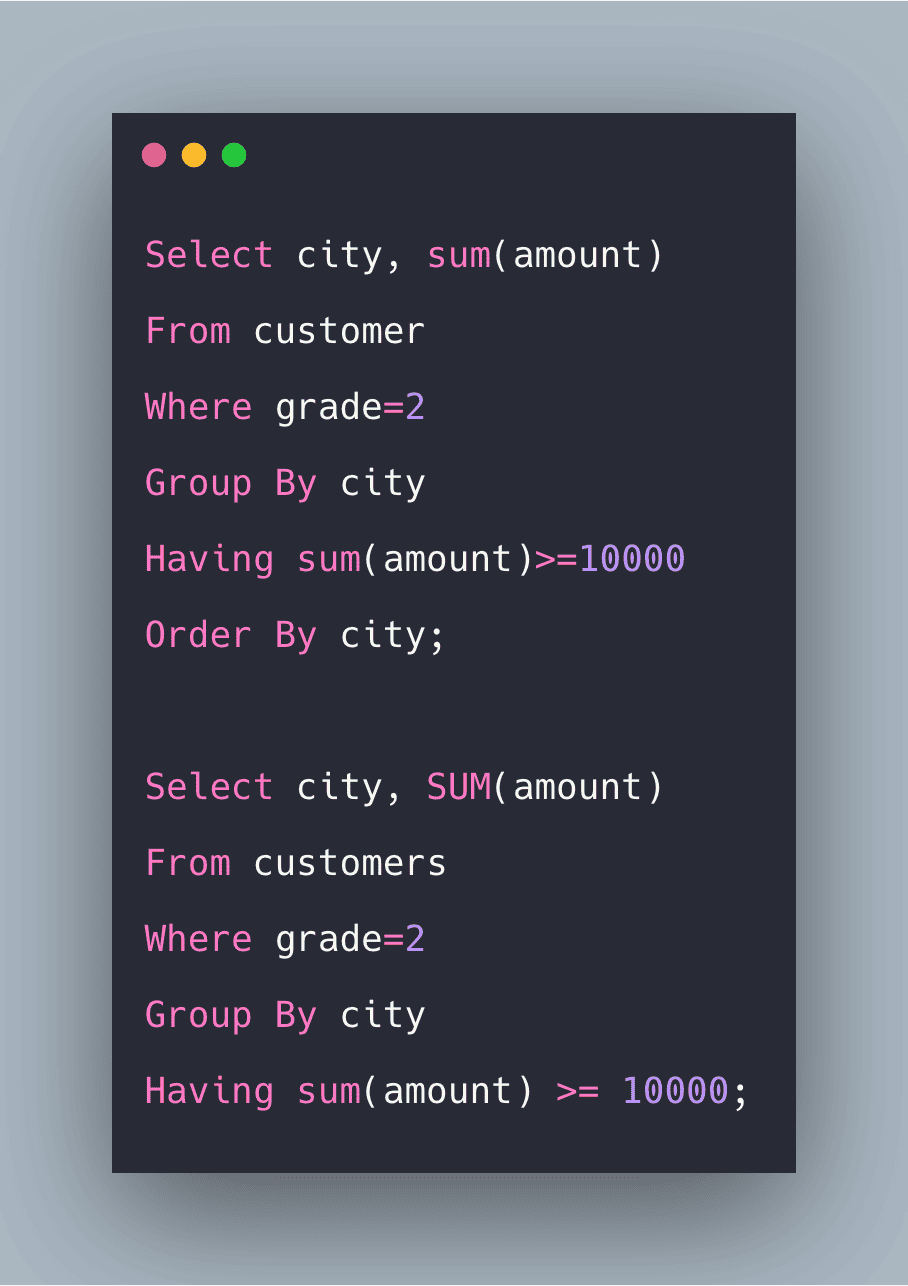SQL Having Clause Example | SQL Having Tutorial is today’s topic. The SQL HAVING clause is used in the combination with a GROUP BY CLAUSE to restrict the groups of returned rows to only those whose condition is TRUE. The HAVING clause was added to SQL because the WHERE keyword could not be used with aggregate functions.

SQL Having Clause Example

SQL HAVING filters the records that work on summarized GROUP BY results. The HAVING applies to the summarized group records, whereas WHERE applies to the individual records. Only the groups that meet a HAVING criteria will be returned. HAVING requires that the GROUP BY clause is present. WHERE and HAVING can be in a same query.

Syntax

``````SELECT Expressions, aggregate functions(aggregate_expression)
FROM TABLE
WHERE CONDITION
GROUP BY COLUMN
HAVING CONDITION;``````

PARAMETERS

Expressions: Expressions that are not encapsulated within the aggregate function and must be included in the GROUP BY Clause near the end of an SQL statement.

Aggregate function: Sum, count, Min, Max are some of the aggregate functions.

Aggregate Expressions: It is the column expression used in an aggregate function.

Table: Name of the table.

Where Condition: Where condition Is used for the records to be selected.

Group By: It is a keyword used for grouping some rows based on the same values.

Having Keyword: This is the further condition applied only to an aggregated results to restrict the groups of the returned rows. Only those groups whose condition evaluates to be TRUE will be included in the result set.

Let’s clear with an example:

Consider the following Table.

CUSTOMERS

 CUST_CODE CUST_NAME CITY GRADE AMOUNT 101 SHUBH KOLKATA 1 10000 201 SOURAV KOLKATA 1 10000 301 KISHAN PATNA 2 20000 401 DILEEP ALLAHABAD 2 30000 501 SHRUTI PATNA 3 40000

In this, we are going to use the aggregate function count. See the following query.

QUERY

``````Select city,count(grade)
From customers
Group By city
``````

See the following output.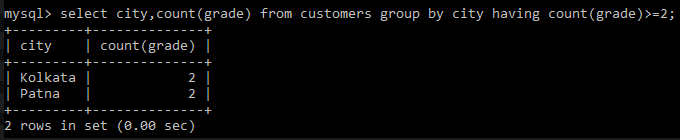Explanation

In the above example, we have displayed the total no of employees having similar grades greater than 2, and their city groups data.

SQL Having With Aggregate MIN

In this example, we are going to use the aggregate function MIN.

QUERY

``````Select city, MIN(amount) as “LESS AMOUT”
From customers
Group By city
Having MIN(amount)>10000;
``````

See the following output.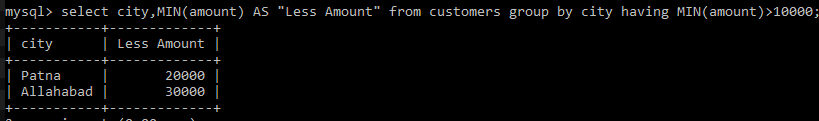Explanation

In this example, we have displayed the Min amount a customer has belonging to the respective city.

SQL Having With Aggregate SUM

In this example, we are going to use an aggregate function SUM.

QUERY

``````Select city, SUM(amount)
From customers
Group By city
Having sum(Amount) >= 10000;
``````

See the output.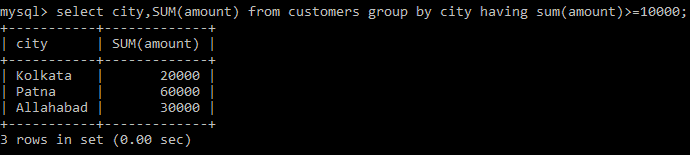Explanation

In this example, we have displayed the total sum of the number of customers belonging to the same city.

SQL Having With Aggregate MAX

In this example, we are going to use the aggregate function MAX.

QUERY

``````Select city, MAX(amount)
From customers
Group By city
Having MAX(amount)<20000;
``````

OUTPUT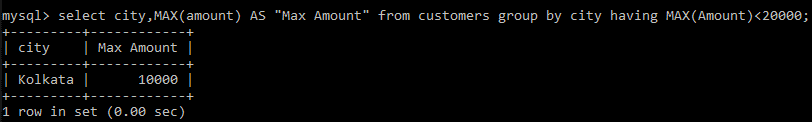Explanation

In this example, we have displayed the max amount from customers grouped by their cities.

SQL Having With Aggregate WHERE

In this example, we are going to use the where keyword.

QUERY

``````Select city, SUM(amount)
From customers
Group By city
Having sum(amount) >= 10000;
``````

OUTPUT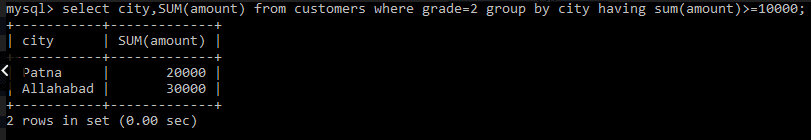In this example, we have displayed the total sum of amount whose grades are 2 and are grouped by their city and also having a total sum greater than equal to 10000.

SQL Having With Aggregate Order By

In this example, we are going to use the ORDER BY keyword.

QUERY

``````Select city, sum(amount)
From customer
Group By city
Having sum(amount)>=10000
Order By city;
``````

OUTPUT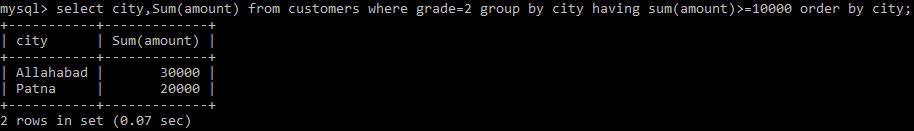Explanation

In this example, we have displayed the total sum of amount whose grades are 2 and are grouped by their city and also having a total sum greater than equal to 10000 followed by order by, which have displayed the city in ascending order as ASC is executed by default.

When WHERE statement, GROUP BY, and HAVING clauses are used together in the SELECT statement, the WHERE clause is processed first and then rows that are returned after a WHERE clause is executed are grouped based on a GROUP BY clause. Finally, any conditions on that group functions in a HAVING clause are applied to the grouped rows before the final output is displayed.

So, we have seen SQL Having with WHERE clause, GROUP BY, ORDER BY and all the aggregate functions.

Finally, SQL Having Clause Example | SQL Having Tutorial is over.